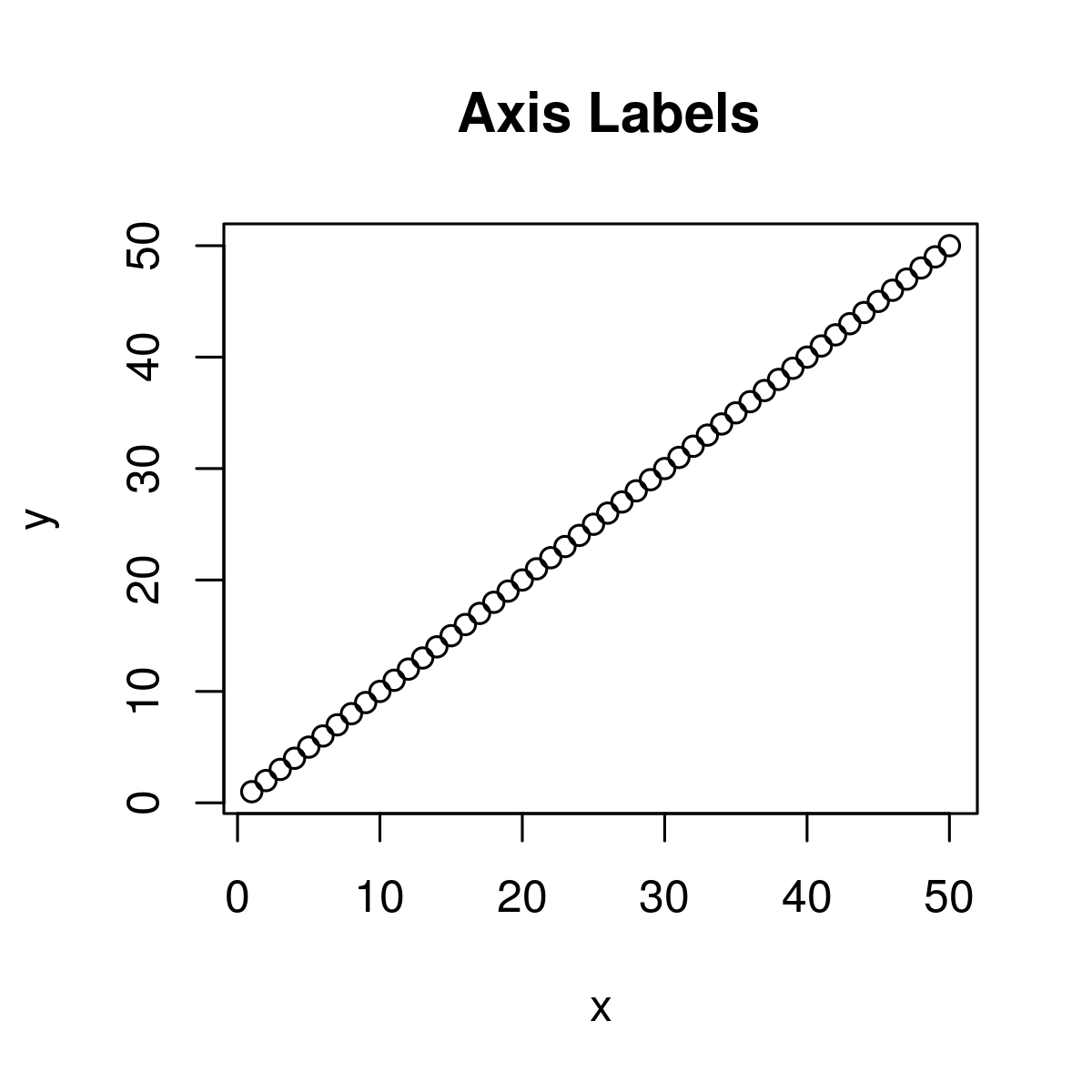# Compute a Sequence of Equally Spaced Round values in R Programming – pretty() Function

• Last Updated : 23 Jun, 2020

`pretty()` function in R Language is used to decide sequence of equally spaced round values.

Syntax: pretty(x, n)

Parameters:
x: It is defined as vector data
n: length of resultant vector

Returns: data vector of equal length interval

Example 1:

 `# Create example vector``# Apply pretty function to vector``x <- 1:50                                 ``pretty``(x)                                     `

Output:

```  0 10 20 30 40 50
```

Example 2:

 `# Create example vector``x <- 1:50     `` ` `# Apply pretty function to vector                            ``pretty``(x, n = 10)                   `

Output:

```
  0  5 10 15 20 25 30 35 40 45 50
```

Here n = 10 states that the interval vector consisting of ten intervals, i.e. n+1 elements.

Example 3:

 `# Create example vectors``x <- 1:50 ``y <- 1:50   `` ` `# Create plot of two vectors                       ``plot``(x, y,        ``    ` `     ``# Default axis labels                     ``     ``main = ``" Axis Labels"``)             `

Output:My Personal Notes arrow_drop_up